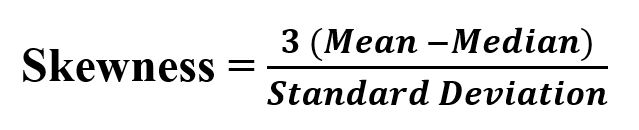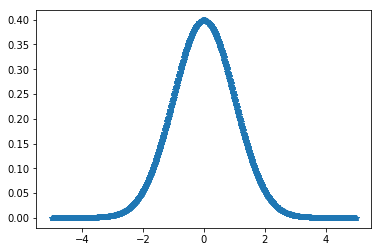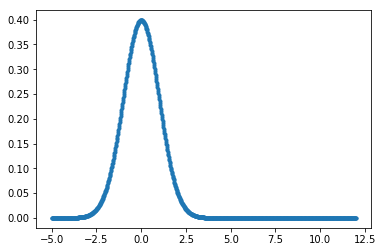Skip to content
Related Articles
scipy stats.skew() | Python
• Last Updated : 11 Feb, 2019

`scipy.stats.skew(array, axis=0, bias=True)` function calculates the skewness of the data set.

```skewness = 0 : normally distributed.
skewness > 0 : more weight in the left tail of the distribution.
skewness < 0 : more weight in the right tail of the distribution.
```

Its formula –Parameters :
array : Input array or object having the elements.
axis : Axis along which the skewness value is to be measured. By default axis = 0.
bias : Bool; calculations are corrected for statistical bias, if set to False.

Returns : Skewness value of the data set, along the axis.

Code #1:

 `# Graph using numpy.linspace() ``# finding Skewness`` ` `from` `scipy.stats ``import` `skew``import` `numpy as np ``import` `pylab as p `` ` `x1 ``=` `np.linspace( ``-``5``, ``5``, ``1000` `)``y1 ``=` `1.``/``(np.sqrt(``2.``*``np.pi)) ``*` `np.exp( ``-``.``5``*``(x1)``*``*``2`  `)`` ` `p.plot(x1, y1, ``'*'``)`` ` `print``( ``'\nSkewness for data : '``, skew(y1))`

Output :

```Skewness for data : 1.1108237139164436
```

Code #2:

 `# Graph using numpy.linspace() ``# finding Skewness`` ` ` ` `from` `scipy.stats ``import` `skew``import` `numpy as np ``import` `pylab as p `` ` `x1 ``=` `np.linspace( ``-``5``, ``12``, ``1000` `)``y1 ``=` `1.``/``(np.sqrt(``2.``*``np.pi)) ``*` `np.exp( ``-``.``5``*``(x1)``*``*``2`  `)`` ` `p.plot(x1, y1, ``'.'``)`` ` `print``( ``'\nSkewness for data : '``, skew(y1))`

Output :

```Skewness for data : 1.917677776148478
```

Code #3: On Random data

 `# finding Skewness`` ` `from` `scipy.stats ``import` `skew``import` `numpy as np `` ` `# random values based on a normal distribution``x ``=` `np.random.normal(``0``, ``2``, ``10000``)`` ` `print` `(``"X : \n"``, x)`` ` `print``(``'\nSkewness for data : '``, skew(x))`

Output :

```X :
[ 0.03255323 -6.18574775 -0.58430139 ...  3.22112446  1.16543279
0.84083317]

Skewness for data :  0.03248837584866293
```

Attention geek! Strengthen your foundations with the Python Programming Foundation Course and learn the basics.

To begin with, your interview preparations Enhance your Data Structures concepts with the Python DS Course. And to begin with your Machine Learning Journey, join the Machine Learning – Basic Level Course

My Personal Notes arrow_drop_up